Computational & Technology Resources
an online resource for computational,
engineering & technology publications
Civil-Comp Proceedings
ISSN 1759-3433
CCP: 84
PROCEEDINGS OF THE FIFTH INTERNATIONAL CONFERENCE ON ENGINEERING COMPUTATIONAL TECHNOLOGY
Edited by: B.H.V. Topping, G. Montero and R. Montenegro
Paper 19

Analysis of the Numerical Methods for a Fractional Dirac Equation

S. Jiménez1 and Y.F. Tang2

1Department of Applied Mathematics for Information Technology, E.T.S.I. Telecommunication, Polytechnic University of Madrid, Spain
2State Key Laboratory of Scientific and Engineering Computing, Chinese Academy of Sciences, Beijing, China

Full Bibliographic Reference for this paper
S. Jiménez, Y.F. Tang, "Analysis of the Numerical Methods for a Fractional Dirac Equation", in B.H.V. Topping, G. Montero, R. Montenegro, (Editors), "Proceedings of the Fifth International Conference on Engineering Computational Technology", Civil-Comp Press, Stirlingshire, UK, Paper 19, 2006. doi:10.4203/ccp.84.19
Keywords: fractional equation, Dirac equation, numerical analysis.

Summary
Lately, the fractional Dirac equation has been considered as an interpolation from the inside between the classical Heat Equation and the Wave Equation [1,2]. In this work we build a suitable numerical scheme to simulate a one-dimensional fractional Dirac equation of the form: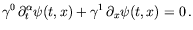(43)

In order to build our numerical scheme, we start using the ideas presented in  for a system that depends on a single variable, we will then extend the ideas to the fractional equation. We start considering a fractional equation of the form: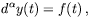(44)

where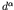is the fractional Caputo derivative of order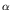with lower limit 0. If we define: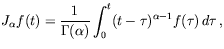(45)

the equation is equivalent to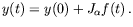(46)

For this equation we have build two first order schemes. Combining both, we can build a second order scheme: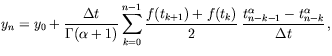(47)

with local truncation error: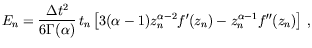(48)

and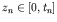. We have used this scheme to represent the fractional time derivative of the solution and a second order scheme for the spatial derivative. The Dirac equation can be expressed in a form similar to (44):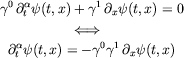(49)

and from here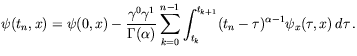(50)

Denoting the spatial index by subscripts and the temporal one by superscripts, we have the numerical scheme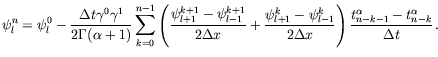(51)

The local truncation error can be written as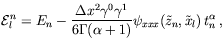(52)

for some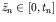and whereis the error given previously, replacing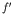by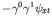and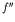by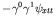. The scheme is locally of order 2 in both mesh parameters and is an implicit scheme since the equation couples three values of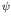at the highest time-level n.

We show that, under reasonable boundary and initial conditions, the errors at each time-step are bounded by an expression that goes to zero as the mesh sizes go to zero, keeping both a finite fixed time and a spatial length. The scheme is thus convergent.

References
1
A.A. Kilbas, T. Pierantozzi, J.J. Trujillo and L. Vázquez. "On the Solution of Fractional Evolution Equations", Journal of Physics A, 37, 3271-3283 (2004). doi:10.1088/0305-4470/37/9/015
2
T. Pierantozzi and L. Vázquez, "An interpolation between the wave and diffusion equations through the fractional evolution equations Dirac like", Journal of Mathematical Physics, 46, 113512-113523 (2005). doi:10.1063/1.2121167
3
G. Turchetti, D. Usero and L. Vázquez, "Hamiltonian systems with fractional time derivative", Tamsui Oxford Journal of Mathematical Sciences, Vol 18 (1), 31-44 (2002).

purchase the full-text of this paper (price £20)